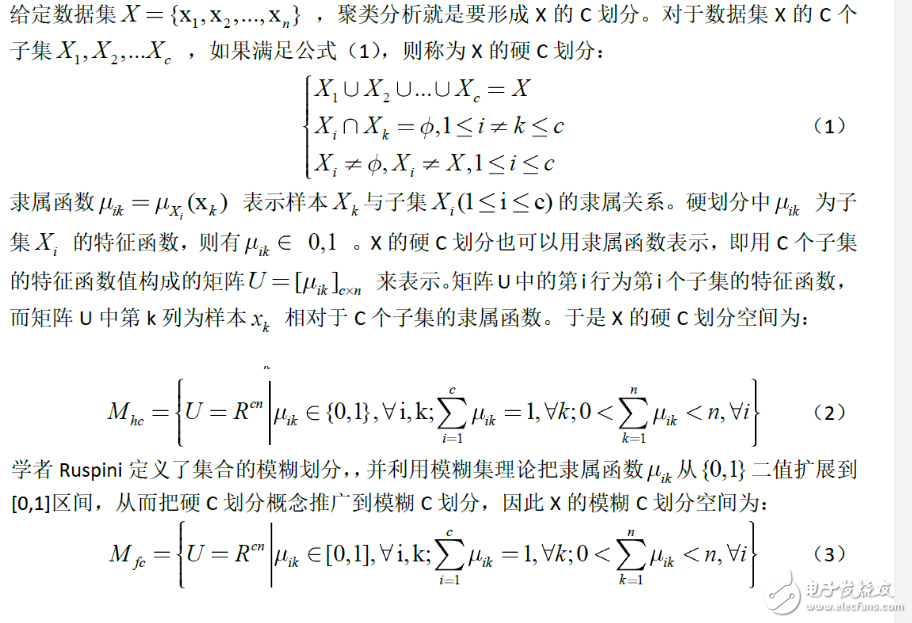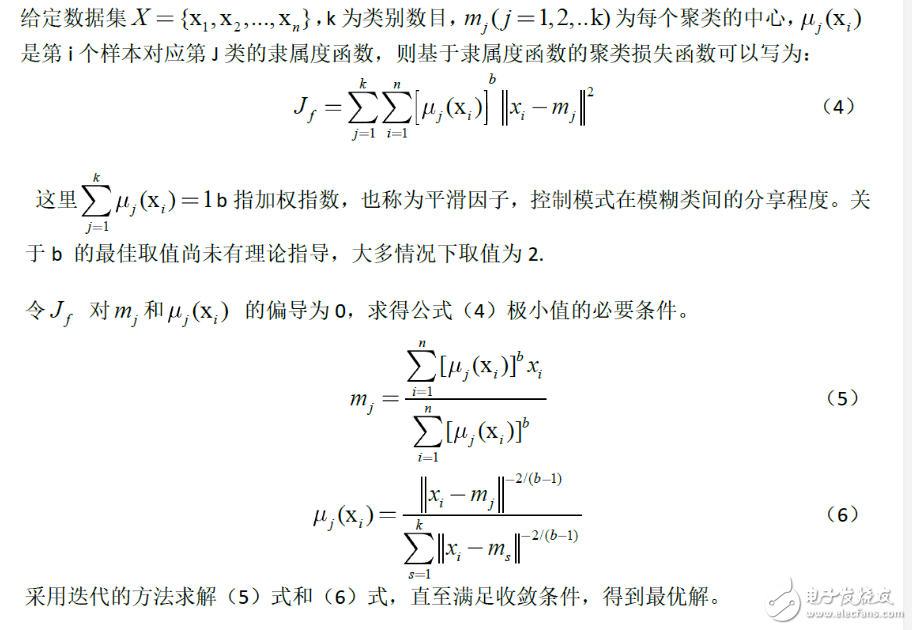• 2021-04-22 00:58:17

应用背景数学系本科毕业设计中有不少是与模糊数学相关的,我的题目是模糊聚类分析的有效性问题初探,FCM算法是我论文中不可或缺的部分,在此我将FCM算法的两种迭代形式的MATLAB代码写于下,也许有的同学会用得着

关键技术

模糊C均值聚类(FCM)，即众所周知的模糊ISODATA，是用隶属度确定每个数据点属于某个聚类的程度的一种聚类算法。1973年，Bezdek提出了该算法，作为早期硬C均值聚类(HCM)方法的一种改进。

FCM把n个向量xi(i=1,2,…,n)分为c个模糊组，并求每组的聚类中心，使得非相似性指标的价值函数达到最小。FCM与HCM的主要区别在于FCM用模糊划分，使得每个给定数据点用值在0，1间的隶属度来确定其属于各个组的程度。与引入模糊划分相适应，隶属矩阵U允许有取值在0，1间的元素。不过，加上归一化规定，一个数据集的隶属度的和总等于1.

FCM的价值函数(或目标函数)就是所有个点隶属度乘以该点与中心的欧氏距离之和。

在批处理方式运行时，FCM用下列步骤确定聚类中心ci和隶属矩阵U：

步骤1：用值在0，1间的随机数初始化隶属矩阵U，使其满足式(6.9)中的约束条件

步骤2：用式(6.12)计算c个聚类中心ci，i=1,…,c。

步骤3：根据式(6.10)计算价值函数。如果它小于某个确定的阀值，或它相对上次价值函数值的改变量小于某个阀值，则算法停止。

步骤4：用(6.13)计算新的U矩阵。返回步骤2。

上述算法也可以先初始化聚类中心，然后再执行迭代过程。由于不能确保FCM收敛于一个最优解。算法的性能依赖于初始聚类中心。因此，我们要么用另外的快速算法确定初始聚类中心，要么每次用不同的初始聚类中心启动该算法，多次运行FCM。

迭代函数：

更多相关内容
• 聚类分割算法是图像处理中的基本操作，文件中包含了使用模糊C-均值实现聚类分割的算法原理以及MAtlab源程序 聚类分割算法是图像处理中的基本操作，文件中包含了使用模糊C-均值实现聚类分割的算法原理以及MAtlab源...
• matlab实现模糊C均值聚类，附带包含600个2维数据的数据集，可视化展示结果。数据集有3类，分别分布在第一、二 三象限。
• 亲测可用！图像模糊C均值聚类分割matlab代码，聚类分割后显示图像。 仅需要自己修改读图路径。
• 简单的模糊c-均值聚类matlab程序 希望对大家能够有所帮助
• 针对模糊C均值聚类算法(FCM)存在对初始聚类中心敏感，易陷入局部最优解的不足，将改进的粒子群聚类算法与FCM算法相结合，提出了一种基于粒子群优化的模糊C均值聚类算法。该算法对粒子群初始化空间及粒子移动最大速度...
• 使用基于模糊 c 均值聚类的机器学习对 2 类和 3 类问题进行一维矩阵分类。 它还包含一个基于矩阵的 AND 门示例和大小为 12 和 3 的输入样本
• 模糊C均值聚类算法实现数据挖掘的matlab源代码
• function [center, U, obj_fcn] = FCMClust(data, cluster_n, options)% FCMClust.m 采用模糊C均值对数据集data聚为cluster_n类%% 用法：% 1. [center,U,obj_fcn] = FCMClust(Data,N_cluster,options);% 2. [center,...

function [center, U, obj_fcn] = FCMClust(data, cluster_n, options)

% FCMClust.m 采用模糊C均值对数据集data聚为cluster_n类

%

% 用法：

% 1. [center,U,obj_fcn] = FCMClust(Data,N_cluster,options);

% 2. [center,U,obj_fcn] = FCMClust(Data,N_cluster);

%

% 输入：

% data ---- nxm矩阵,表示n个样本,每个样本具有m的维特征值

% N_cluster ---- 标量,表示聚合中心数目,即类别数

% options ---- 4x1矩阵，其中

% options(1): 隶属度矩阵U的指数，>1 (缺省值: 2.0)

% options(2): 最大迭代次数 (缺省值: 100)

% options(3): 隶属度最小变化量,迭代终止条件 (缺省值: 1e-5)

% options(4): 每次迭代是否输出信息标志 (缺省值: 1)

% 输出：

% center ---- 聚类中心

% U ---- 隶属度矩阵

% obj_fcn ---- 目标函数值

% Example:

% data = rand(100,2);

% [center,U,obj_fcn] = FCMClust(data,2);

% plot(data(:,1), data(:,2),'o');

% hold on;

% maxU = max(U);

% index1 = find(U(1,:) == maxU);

% index2 = find(U(2,:) == maxU);

% line(data(index1,1),data(index1,2),'marker','*','color','g');

% line(data(index2,1),data(index2,2),'marker','*','color','r');

% plot([center([1 2],1)],[center([1 2],2)],'*','color','k')

% hold off;

if nargin ~= 2 & nargin ~= 3, %判断输入参数个数只能是2个或3个

error('Too many or too few input arguments!');

end

data_n = size(data, 1); % 求出data的第一维(rows)数,即样本个数

in_n = size(data, 2); % 求出data的第二维(columns)数，即特征值长度

% 默认操作参数

default_options = [2; % 隶属度矩阵U的指数

100; % 最大迭代次数

1e-5; % 隶属度最小变化量,迭代终止条件

1]; % 每次迭代是否输出信息标志

if nargin == 2,

options = default_options;

else %分析有options做参数时候的情况

% 如果输入参数个数是二那么就调用默认的option;

if length(options) < 4, %如果用户给的opition数少于4个那么其他用默认值;

tmp = default_options;

tmp(1:length(options)) = options;

options = tmp;

end

% 返回options中是数的值为0(如NaN),不是数时为1

nan_index = find(isnan(options)==1);

%将denfault_options中对应位置的参数赋值给options中不是数的位置.

options(nan_index) = default_options(nan_index);

if options(1) <= 1, %如果模糊矩阵的指数小于等于1

error('The exponent should be greater than 1!');

end

end

%将options 中的分量分别赋值给四个变量;

expo = options(1); % 隶属度矩阵U的指数

max_iter = options(2); % 最大迭代次数

min_impro = options(3); % 隶属度最小变化量,迭代终止条件

display = options(4); % 每次迭代是否输出信息标志

obj_fcn = zeros(max_iter, 1); % 初始化输出参数obj_fcn

U = initfcm(cluster_n, data_n); % 初始化模糊分配矩阵,使U满足列上相加为1,

% Main loop 主要循环

for i = 1:max_iter,

%在第k步循环中改变聚类中心ceneter,和分配函数U的隶属度值;

[U, center, obj_fcn(i)] = stepfcm(data, U, cluster_n, expo);

if display,

fprintf('FCM:Iteration count = %d, obj. fcn = %f\n', i, obj_fcn(i));

end

% 终止条件判别

if i > 1,

if abs(obj_fcn(i) - obj_fcn(i-1)) < min_impro,

break;

end,

end

end

iter_n = i; % 实际迭代次数

obj_fcn(iter_n+1:max_iter) = [];

% 子函数

function U = initfcm(cluster_n, data_n)

% 初始化fcm的隶属度函数矩阵

% 输入:

% cluster_n ---- 聚类中心个数

% data_n ---- 样本点数

% 输出：

% U ---- 初始化的隶属度矩阵

U = rand(cluster_n, data_n);

col_sum = sum(U);

U = U./col_sum(ones(cluster_n, 1), :);

% 子函数

function [U_new, center, obj_fcn] = stepfcm(data, U, cluster_n, expo)

% 模糊C均值聚类时迭代的一步

% 输入：

% data ---- nxm矩阵,表示n个样本,每个样本具有m的维特征值

% U ---- 隶属度矩阵

% cluster_n ---- 标量,表示聚合中心数目,即类别数

% expo ---- 隶属度矩阵U的指数

% 输出：

% U_new ---- 迭代计算出的新的隶属度矩阵

% center ---- 迭代计算出的新的聚类中心

% obj_fcn ---- 目标函数值

mf = U.^expo; % 隶属度矩阵进行指数运算结果

center = mf*data./((ones(size(data, 2), 1)*sum(mf'))'); % 新聚类中心(5.4)式

dist = distfcm(center, data); % 计算距离矩阵

obj_fcn = sum(sum((dist.^2).*mf)); % 计算目标函数值 (5.1)式

tmp = dist.^(-2/(expo-1));

U_new = tmp./(ones(cluster_n, 1)*sum(tmp)); % 计算新的隶属度矩阵 (5.3)式

% 子函数

function out = distfcm(center, data)

% 计算样本点距离聚类中心的距离

% 输入：

% center ---- 聚类中心

% data ---- 样本点

% 输出：

% out ---- 距离

out = zeros(size(center, 1), size(data, 1));

for k = 1:size(center, 1), % 对每一个聚类中心

% 每一次循环求得所有样本点到一个聚类中心的距离

out(k, :) = sqrt(sum(((data-ones(size(data,1),1)*center(k,:)).^2)',1));

end

展开全文• 这是一个基于粒子群优化算法的模糊c均值聚类源码（matlab) 这是一个基于粒子群优化算法的模糊c均值聚类源码（matlab)
• function [center, U, obj_fcn] = FCMClust(data, cluster_n, options)% FCMClust.m 采用模糊C均值对数据集data聚为cluster_n类% 用法：% 1. [center,U,obj_fcn] = FCMClust(Data,N_cluster,options);% 2. [center,U...

function [center, U, obj_fcn] = FCMClust(data, cluster_n, options)

% FCMClust.m 采用模糊C均值对数据集data聚为cluster_n类

% 用法：

% 1. [center,U,obj_fcn] = FCMClust(Data,N_cluster,options);

% 2. [center,U,obj_fcn] = FCMClust(Data,N_cluster);

% 输入：

% data ---- nxm矩阵,表示n个样本,每个样本具有m的维特征值

% N_cluster ---- 标量,表示聚合中心数目,即类别数

% options ---- 4x1矩阵，其中

% options(1): 隶属度矩阵U的指数，>1 (缺省值: 2.0)

% options(2): 最大迭代次数(缺省值: 100)

% options(3): 隶属度最小变化量,迭代终止条件(缺省值: 1e-5)

% options(4): 每次迭代是否输出信息标志(缺省值: 1)

% 输出：

% center ---- 聚类中心

% U ---- 隶属度矩阵

% obj_fcn ---- 目标函数值

% Example:

% data = rand(100,2);

% [center,U,obj_fcn] = FCMClust(data,2);

% plot(data(:,1), data(:,2),'o');

% hold on;

% maxU = max(U);

% index1 = find(U(1,:) == maxU);

% index2 = find(U(2,:) == maxU);

% line(data(index1,1),data(index1,2),'marker','*','color','g');

% line(data(index2,1),data(index2,2),'marker','*','color','r');

% plot([center([1 2],1)],[center([1 2],2)],'*','color','k')

% hold off; %%%%%%%%%%%%%%%%%%%%%%%%%%%%%%%%%%%%%%%%%%%%%%% %%%%%%%%%%%%%%%%%%%%%%%%%%%% %%%%%%%%%%%%%%%%%%%%%%%%%%%%%%%%%%%%%%%%%%%%%%% %%%%%%%%%%%%%%%%%%%%%%%%%%%%

if nargin ~= 2 & nargin ~= 3, %判断输入参数个数只能是2个或3个

error('Too many or too few input arguments!');

end

data_n = size(data, 1); % 求出data的第一维(rows)数,即样本个数

in_n = size(data, 2); % 求出data的第二维(columns)数，即特征值长度

% 默认操作参数

default_options = [2; % 隶属度矩阵U的指数

100; % 最大迭代次数

1e-5; % 隶属度最小变化量,迭代终止条件

展开全文• 自己写的模糊C均值聚类算法，也就是FCM，用matlab写的，希望能帮助大家学习
• c均值聚类算法matlab代码fcm_m 这是matlab代码中FCM聚类方法的一些变体。 您可以参考以下文章以进一步了解：“一种鲁棒的模糊局部信息C均值聚类算法”
• 描述聚类分析是多元统计分析的一种，也是无监督模式识别的一个重要分支，在模式分类 图像处理和模糊规则处理等众多领域中获得最广泛的应用。它把一个没有类别标记的样本按照某种准则划分为若干子集，使相似的样本尽...

描述

聚类分析是多元统计分析的一种，也是无监督模式识别的一个重要分支，在模式分类 图像处理和模糊规则处理等众多领域中获得最广泛的应用。它把一个没有类别标记的样本按照某种准则划分为若干子集，使相似的样本尽可能归于一类，而把不相似的样本划分到不同的类中。硬聚类把每个待识别的对象严格的划分某类中，具有非此即彼的性质，而模糊聚类建立了样本对类别的不确定描述，更能客观的反应客观世界，从而成为聚类分析的主流。

模糊聚类算法是一种基于函数最优方法的聚类算法，使用微积分计算技术求最优代价函数，在基于概率算法的聚类方法中将使用概率密度函数，为此要假定合适的模型，模糊聚类算法的向量可以同时属于多个聚类，从而摆脱上述问题。

模糊聚类分析算法大致可分为三类

1)分类数不定，根据不同要求对事物进行动态聚类，此类方法是基于模糊等价矩阵聚类的，称为模糊等价矩阵动态聚类分析法。

2)分类数给定，寻找出对事物的最佳分析方案，此类方法是基于目标函数聚类的，称为模糊C均值聚类。

3)在摄动有意义的情况下，根据模糊相似矩阵聚类，此类方法称为基于摄动的模糊聚类分析法

模糊的c均值聚类算法：-------- 一种模糊聚类算法，是k均值聚类算法的推广形式，隶属度取值为［0 1］区间内的任何一个数，提出的基本根据是“类内加权误差平方和最小化”准则；

模糊C 均值聚类算法(FCM，Fuzzy c-means) 是从硬C 均值聚类算法发展而来(HCM，Hardc-means )。

硬C划分和模糊C 划分FCM算法原理FCM 算法步骤

给定聚类类别数C，设定迭代收敛条件，初始化各个聚类中心;

(1)重复下面的运算，直到各个样本的隶属度值稳定：

(2)用当前的聚类中心根据公式(6) 计算隶属度函数;

A.用当前的隶属度函数根据公式(5) 重新计算各个聚类的中心。

B.当算法收敛时，就得到了各类的聚类中心和各个样本对于各类的隶属度值，从而完成了模糊聚类划分。

算法实现

·采用VC++进行编写

文档的读取

#include “data.h”

//函数定义

{

double**p=new double* ［row］;

ifstreaminfile;

infile.open(name，ios：：in);

for(inti=0;i《row;i++)

{

p［i］=newdouble［col］;

for(intj=0;j《col;j++)

{

infile》》p［i］［j］;

}

}

infile.close();

cout《《“成功读取数据文件：”《《name《《“！\n”;

returnp;

//释放内存

for(i=0;i《row;i++)

{

delete［］p［i］;

}

delete［］p;

}

文档的保存

#include “data.h”

void DataSave(double**data，int row，intcol，char*name)

{

inti，j;

ofstreamoutfile;

//打开文件，输出数据

outfile.open(name，ios：：out);

outfile.setf(ios：：fixed);

outfile.precision(4);

for(i=0;i《row;i++)

{

for(j=0;j《col;j++)

{

outfile《《data［i］［j］《《“”;

}

outfile《《endl;

}

outfile《《endl《《endl;

outfile.close();

}

数据标准化处理

#include “data.h”

double **Standardize(double **data，introw，int col)

{

inti，j;

double*a=new double［col］; //矩阵每列的最大值

double*b=new double［col］; //矩阵每列的最小值

double*c=new double［row］; //矩阵列元素

for(i=0;i《col;i++)

{

//取出数据矩阵的各列元素

for(j=0;j《row;j++)

{

c［j］=Data［j］［i］;

}

a［i］=c［0］，b［i］=c［0］;

for(j=0;j《row;j++)

{

//取出该列的最大值

if(c［j］》a［i］)

{

a［i］=c［j］;

}

//取出该列的最小值

if(c［j］《b［i］)

{

b［i］=c［j］;

}

}

}

//数据标准化

for(i=0;i《row;i++)

{

for(j=0;j《col;j++)

{

data［i］［j］=(data［i］［j］-b［j］)/(a［j］-b［j］);

}

}

cout《《“完成数据极差标准化处理！\n”;

delete［］a;

delete［］b;

delete［］c;

returndata;

}

生成样本虑属矩阵

#include “data.h”

void Initialize(double **u， int k， introw)

{

inti，j;

//初始化样本隶属度矩阵

srand(time(0));

for(i=0;i《k;i++)

{

for(j=0;j《row;j++)

{

u［i］［j］=(double)rand()/RAND_MAX;//得到一个小于1的小数隶属度

}//rand()函数返回0和RAND_MAX之间的一个伪随机数

}

}

数据归一化处理

#include “data.h”

void Normalize(double **u，int k，intcol)

{

inti，j;

double*sum=new double［col］;

//矩阵U的各列元素之和

for(j=0;j《col;j++)

{

doubledj=0;

for(i=0;i《k;i++)

{

dj=dj+U［i］［j］;

sum［j］=dj;//隶属度各列之和

}

}

for(i=0;i《k;i++)

{

for(j=0;j《col;j++)

{

u［i］［j］=U［i］［j］/sum［j］;

}//规一化处理(每列隶属度之和为1)

}

}

迭代过程

#include “data.h”

#include “func.h”//对模糊C均值进行迭代运算，并返回有效性评价函数的值

doubleUpdate(double**u，double**data，double**center，int row，int col， int k)

{

inti，j，t;

double**p=NULL;

for(i=0;i《k;i++)

{

for(j=0;j《row;j++)

{

//模糊指数取2

u［i］［j］=pow(u［i］［j］，2);

}

}

//根据隶属度矩阵计算聚类中心

p=MatrixMul(u，k，row，data，row，col);

for(i=0;i《k;i++)

{

//计算隶属度矩阵每行之和

doublesi=0;

for(j=0;j《row;j++)

{

si+=u［i］［j］;

}

for(t=0;t《col;t++)

{

center［i］［t］=p［i］［t］/si; //类中心

}

}

//计算各个聚类中心i分别到所有点j的距离矩阵dis(i，j)

double*a=new double［col］; //第一个样本点

double*b=new double［col］; //第二个样本点

double**dis=newdouble*［k］; //中心与样本之间距离矩阵

for(i=0;i《k;i++)

{

dis［i］=newdouble［row］;

}

for(i=0;i《k; i++)

{

//聚类中心

for(t=0;t《col; t++)

{

a［t］=center［i］［t］; //暂存类中心

}

//数据样本

for(j=0;j《row; j++)

{

for(t=0;t《col; t++)

{

b［t］=data［j］［t］;//暂存一样本

}

doubled=0;

//中心与样本之间距离的计算

for(t=0;t《col; t++)

{

d+=(a［t］-b［t］)*(a［t］-b［t］); //d为一中心与所有样本的距离的平方和

}

dis［i］［j］=sqrt(d); //距离

}

}

//根据距离矩阵计算隶属度矩阵

for(i=0;i《k;i++)

{

for(j=0;j《row;j++)

{

doubletemp=0;

for(t=0;t《k;t++)

{

//dis［i］［j］依次除以所在列的各元素，加和；

//模糊指数为2.0

temp+=pow(dis［i］［j］/dis［t］［j］，2/(2.0-1));//一个类中心和一个元素的距离平方与

}

u［i］［j］=1/temp;//所有类与该元素距离平方的和的商

}

}

//计算聚类有效性评价函数

doublefunc1=0;

for(i=0;i《k;i++)

{

doublefunc2=0;

for(j=0;j《row;j++)

{

func2+=pow(u［i］［j］，2.0)*pow(dis［i］［j］，2);

}

func1+=func2;

}

doubleobj_fcn=1/(1+func1);

returnobj_fcn;

//内存释放

delete［］a;

delete［］b;

for(i=0;i《k;i++)

{

delete［］dis［i］;

}

delete［］dis;

}

详细过程

#include “data.h”

#include “func.h”

#include “max.h”

//全局变量定义

double **Data; //数据矩阵

double **Center; //聚类中心矩阵

double **U; //样本隶属度矩阵

int m; //样本总数

int n; //样本属性数

int k; //设定的划分类别数

int main()

{

intLab; //数据文件标号

intnum; //算法运行次数

///

cout《《“模糊C均值聚类算法：”《《endl;

cout《《“1-iris.txt; 2-wine.txt; 3-ASD_12_2.txt; 4-ASD_14_2.txt”《《endl;

cout《《“请选择数据集： Lab=”;

cin》》Lab;

cout《《“设定运行次数： mum=”;

cin》》num;

//各次运行结束后的目标函数

double*Index=new double［num］;

//各次运行结束后的聚类正确率

double*R=new double ［num］;

//num次运行的平均目标函数及平均正确率

doubleM_Index=0;

doubleM_R=0;

//FCM聚类算法运行num次，并保存记录与结果

for(inti=0;i《num;i++)

{

intj;

doubleepsilon=1e-4;

inte=0;

intnx=0;

//记录连续无改进次数

intE［200］={0};

if(i》0)

{

cout《《endl《《endl;

cout《《setfill(‘#’)《《setw(10)《《endl;

}

cout《《“第”《《i+1《《“次运行记录：”《《endl;

//读取数据文件

if(Lab==1)

{

m=150;

n=4;

k=3;

}

elseif(Lab==2)

{

m=178;

n=13;

k=3;

}

elseif(Lab==3)

{

m=535;

n=2;

k=12;

}

elseif(Lab==4)

{

m=685;

n=2;

k=14;

}

//数据极差标准化处理

Data=Standardize(Data，m，n);

//聚类中心及隶属度矩阵，内存分配

Center=newdouble*［k］;

U=newdouble *［k］;

for(j=0;j《k;j++)

{

Center［j］=newdouble［n］;

U［j］=newdouble［m］;

}

//隶属度矩阵的初始化

Initialize(U，k， m);

//对隶属度矩阵进行归一化

Normalize(U，k，m);

//历次迭代过程中的目标函数

doubleObjfcn［100］={0};

cout《《“第”《《i+1《《“次运行记录：”《《endl;

cout《《“开始迭代过程！”《《endl;

cout《《“*******************************”《《endl;

//输出精度为小数点后5位

cout.precision(5);

//固定格式

cout.setf(ios：：fixed);

//目标函数连续20代无改进，停止该次聚类迭代过程

while(e《20)

{

nx++;

//聚类迭代过程

Objfcn［nx］=Update(U，Data，Center，m，n，k);

//统计目标函数连续无改进次数e

if(nx》0&& Objfcn［nx］-Objfcn［nx-1］《epsilon )

{

e++;

}

else

{

e=0;

}

E［nx］=e;

}

//输出结果到文件，保存

ofstreamoutfile(“运行记录.txt”，ios：：app);

outfile《《“第”《《i+1《《“次运行记录：”《《endl;

outfile《《“开始迭代过程！”《《endl;

outfile《《“*******************************”《《endl;

outfile.precision(5);

outfile.setf(ios：：fixed);

for(intn1=1;n1《=nx;n1++)

{

cout《《“e［”《《setw(2)《《n1《《“］=”《《setw(2)《《E［n1］《《“ Objfcn［”

《《setw(2)《《n1《《“］=”《《Objfcn［n1］《《“\n”;

//保存数据文件

outfile《《“e［”《《setw(2)《《n1《《“］=”《《setw(2)《《E［n1］《《“ Objfcn［”

《《setw(2)《《n1《《“］=”《《Objfcn［n1］《《“\n”;

}

cout《《endl;

outfile《《endl;

outfile.close();

//本次运行的最大目标函数

Index［i］=Objfcn［nx］;

//保存聚类正确率，输出聚类结果：

R［i］=Result(Lab，U， k， m， i);

//内存释放

for(j=0;j《k;j++)

{

delete［］Center［j］;

delete［］U［j］;

}

delete［］Center;

delete［］U;

}

//统计平均///

doubletemp1=0， temp2=0;

for(i=0;i《num;i++)

{

temp1+=Index［i］;

temp2+=R［i］;

}

//计算各次结果的统计平均

M_Index=(double)temp1/num;

M_R=(double)temp2/num;

cout《《“//”《《endl;

cout《《num《《“次运行，平均聚类正确率： ”《《100*M_R《《“%”《《endl;

//输出精度为小数点后6位

cout.precision(6);

//固定格式

cout.setf(ios：：fixed);

cout《《“平均目标函数：”《《M_Index《《endl;

//统计结果文件保存

ofstreamresultfile(“聚类结果.txt”，ios：：app);

resultfile《《“//”《《endl;

resultfile《《num《《“次运行，平均聚类正确率： ”《《100*M_R《《“%”《《endl;

//输出精度为小数点后6位

resultfile.precision(6);

//固定格式

resultfile.setf(ios：：fixed);

resultfile《《“平均目标函数：”《《M_Index《《endl;

return0;

}

打开APP阅读更多精彩内容

点击阅读全文

展开全文• 聚类分析是多元统计分析的一种，也是无监督模式识别的一个重要分支，在模式分类 图像处理和模糊规则处理等众多领域中获得最广泛的应用。它把一个没有类别标记的样本按照某种准则划分为若干子集，使相似的样本尽可能...
• 利用粒子群优化（PSO）算法全局寻优、快速收敛的特点，结合模糊C-均值（FCM）算法提出一种新的模糊聚类算法。 新算法用PSO算法代替了FCM算法的基于梯度下降的迭代过程，使算法具有很强的全局搜索能力，很大程度上...
• 提出了一种快速且鲁棒的模糊c-means聚类算法，即FRFCM。 FRFCM 能够分割灰度和彩色图像并提供出色的分割结果。
• data=[1739.94 1675.15 2395.96 373.3 3087.05 2429.47 1756.77 1652 1514.98 864.45 1647.31 2665.... title('模糊C均值聚类分析图'); xlabel('第一特征坐标'); ylabel('第二特征坐标'); zlabel('第三特征坐标');矩阵
• 模糊c-均值(FCM)聚类算法在matlab中实现,已测试通过
• FCM+KFCM模糊C均值聚类分析算法，matlab代码，有界面，有图片，运行正常，附pdf说明文档
• 遗传算法改进的模糊C-均值聚类MATLAB源码.doc
• c-means 和模糊 c-means 聚类是两种非常流行的图像分割算法。 虽然它们的实现很简单，但如果天真地实现，它将导致执行时间和内存消耗的大量开销。 尽管对于小型 2D 图像可以忽略这些缺陷，但对于大型 3D 数据集，...
• 模糊Ｃ均值聚类,模糊c均值聚类算法的优缺点,matlab源码
• matlab基于局部信息的模糊C均值聚类算法（FLICM）实现图像分割.md
• 采用C均值聚类算法对男女生样本数据中的身高、体重2个特征进行聚类分析，考察不同的类别初始值以及类别数对聚类结果的影响，并以友好的方式图示化结果。
• 均值聚类算法中的聚类权重和组局部特征权重学习”，应用软计算，2021 （提交）。 为算法的所有步骤编写注释以更好地理解代码。 此外，为了便于运行，还实现了一个演示，它是通过导入数据和其他必要的算法参数来运行......

# 模糊c均值聚类matlabmatlab 订阅